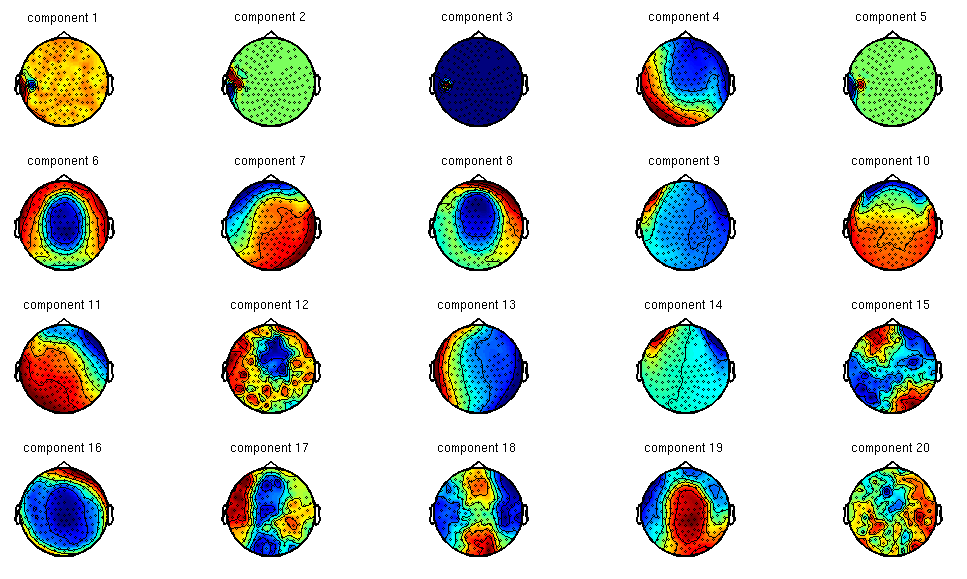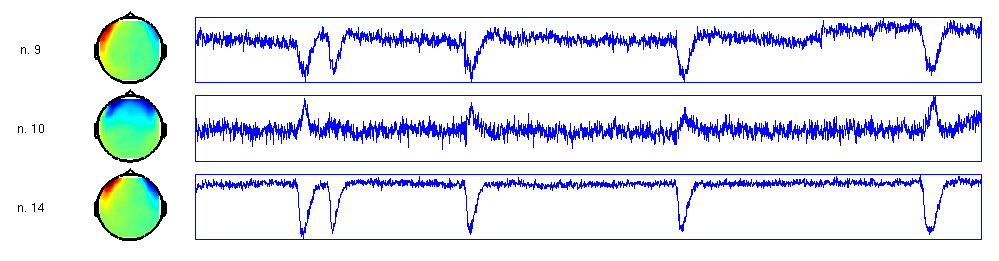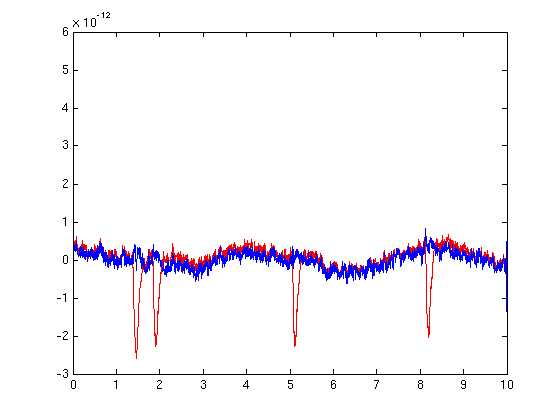# Use independent component analysis (ICA) to remove EOG artifacts

## Description

This script demonstrates how you can use ICA for cleaning the EOG artifacts from your MEG data. It consists of three steps:

1. decomposition of the MEG data
2. identifying the components that reflect eye artifacts
3. removing those components and backprojecting the data

## Example dataset

You can run the code below on your own data. Alternatively, try with the ArtifactMEG.zip example MEG dataset. This dataset was acquired continuously with trials of 10 seconds. All figures in this example script are based on these data.

To load this dataset into MATLAB and preprocess with FieldTrip, use:

``````% preprocessing of example dataset
cfg = [];
cfg.dataset            = 'ArtifactMEG.ds';
cfg.trialdef.eventtype = 'trial';
cfg = ft_definetrial(cfg);

cfg.channel            = 'MEG';
cfg.continuous         = 'yes';
data = ft_preprocessing(cfg);

% downsample the data to speed up the next step
cfg = [];
cfg.resamplefs = 300;
cfg.detrend    = 'no';
data = ft_resampledata(cfg, data);
``````

## ICA decomposition

After reading in the preprocessed data into memory in FieldTrip format, you can continue with decomposing it in independent components.

``````% perform the independent component analysis (i.e., decompose the data)
cfg        = [];
cfg.method = 'runica'; % this is the default and uses the implementation from EEGLAB

comp = ft_componentanalysis(cfg, data);
``````

Note that this is a time-consuming step. The output “comp” structure resembles the input raw data structure, i.e. it contains a time course for each component and each trial. Furthermore, it contains the spatial mixing matrix. In principle you can continue analyzing the data on the component level by doing

``````  cfg = [];
cfg = ...
freq = ft_freqanalysis(cfg, comp);
``````

or

``````  cfg = [];
cfg = ...
timelock = ft_timelockanalysis(cfg, comp);
``````

but for this example we want to analyze the data eventually on the original channel level and only remove the components that represent the artifacts.

## Identify the artifacts

``````% plot the components for visual inspection
figure
cfg = [];
cfg.component = 1:20;       % specify the component(s) that should be plotted
cfg.layout    = 'CTF151.lay'; % specify the layout file that should be used for plotting
cfg.comment   = 'no';
ft_topoplotIC(cfg, comp)
``````

Make sure to plot and inspect all components. Write down the components that contain the eye artifacts. Very important is to know that on subsequent evaluations of the component decomposition result in components that can have a different order. That means that component numbers that you write down do not apply to another run of the ICA decomposition on the same data.The spatial topography of the components aids in interpreting whether a component represents activity from the cortex, or non-cortical physiological activity (muscle, eyes, heart) or even non-physiological activity (line noise and other environmental noise). If you are trained in this type of analysis, you can relatively easily spot the components that represent the eye movements: 9, 14 and 10.

Besides the spatial topography you should inspect the time course of the components, which gives additional information on separating the cortical from the non-cortical contributions to the data.

For further inspection of the time course of the components, use:

``````cfg = [];
cfg.layout = 'CTF151.lay'; % specify the layout file that should be used for plotting
cfg.viewmode = 'component';
ft_databrowser(cfg, comp)
``````

You can browse through the components and the trials. The EOG artifacts can be easily identified in the time course plots, see the figure below for an example.## Remove the artifacts

``````% remove the bad components and backproject the data
cfg = [];
cfg.component = [9 10 14 24]; % to be removed component(s)
data = ft_rejectcomponent(cfg, comp, data)
``````

Compare the data before (red trace) and after (blue trace) the EOG removal - for example trial 4, channel MLF1